###### Improvements in Deep Q-Learning with Python code: Dueling Double DQN, Prioritized Experience Replay, and Fixed Q-targets
2022-09-20###### Handling cyclical features, such as hours in a day, for machine learning pipelines with Python example
2022-09-26

The attention mechanism was introduced to improve the performance of the encoder-decoder model for machine translation. The idea behind the attention mechanism was to permit the decoder to utilize the most relevant parts of the input sequence in a flexible manner, by a weighted combination of all of the encoded input vectors, with the most relevant vectors being attributed to the highest weights.

## The Attention Mechanism

The attention mechanism was introduced by Bahdanau et al. (2014), to address the bottleneck problem that arises with the use of a fixed-length encoding vector, where the decoder would have limited access to the information provided by the input. This is thought to become especially problematic for long and/or complex sequences, where the dimensionality of their representation would be forced to be the same as for shorter or simpler sequences.

Bahdanau et al.’s attention mechanism is divided into the step-by-step computations of the alignment scores, the weights, and the context vector:

1. Alignment scores: The alignment model takes the encoded hidden states,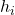, and the previous decoder output,, to compute a score,that indicates how well the elements of the input sequence align with the current output at position, t. The alignment model is represented by a function,, which can be implemented by a feedforward neural network: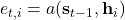1. Weights: The weights, α_t,i are computed by applying a softmax operation to the previously computed alignment scores: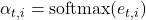1. Context vector: A unique context vector,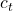, is fed into the decoder at each time step. It is computed by a weighted sum of all, T, encoder hidden states: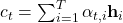Bahdanau et al. implemented an RNN for both the encoder and decoder.  However, the attention mechanism can be re-formulated into a general form that can be applied to any sequence-to-sequence (abbreviated to seq2seq) task, where the information may not necessarily be related in a sequential fashion.

## The General Attention Mechanism

The general attention mechanism makes use of three main components, namely the queries, Q, the keys, K, and the values, V.  If we had to compare these three components to the attention mechanism as proposed by Bahdanau et al., then the query would be analogous to the previous decoder output, while the values would be analogous to the encoded inputs,. In the Bahdanau attention mechanism, the keys and values are the same vectors.

The general attention mechanism then performs the following computations:

1. Each query vector,, is matched against a database of keys to compute a score value. This matching operation is computed as the dot product of the specific query under consideration with each key vector,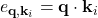1. The scores are passed through a softmax operation to generate the weights: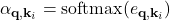1. The generalized attention is then computed by a weighted sum of the value vectors,, where each value vector is paired with a corresponding key:Within the context of machine translation, each word in an input sentence would be attributed to its own query, key, and value vectors. These vectors are generated by multiplying the encoder’s representation of the specific word under consideration, with three different weight matrices that would have been generated during training.

In essence, when the generalized attention mechanism is presented with a sequence of words, it takes the query vector attributed to some specific word in the sequence and scores it against each key in the database. In doing so, it captures how the word under consideration relates to the others in the sequence. Then it scales the values according to the attention weights (computed from the scores), in order to retain focus on those words that are relevant to the query. In doing so, it produces an attention output for the word under consideration.

## The General Attention Mechanism with NumPy and SciPy

In this section, we will explore how to implement the general attention mechanism using the NumPy and SciPy libraries in Python. For simplicity, we will initially calculate the attention for the first word in a sequence of four. We will then generalize the code to calculate an attention output for all four words in matrix form.

Hence, let’s start by first defining the word embeddings of the four different words for which we will be calculating the attention. In actual practice, these word embeddings would have been generated by an encoder, however, for this particular example, we shall be defining them manually.

# encoder representations of four different words
word_1 = array([1, 0, 0])
word_2 = array([0, 1, 0])
word_3 = array([1, 1, 0])
word_4 = array([0, 0, 1])


The next step generates the weight matrices, which we will eventually be multiplying to the word embeddings to generate the queries, keys, and values. Here, we shall be generating these weight matrices randomly, however, in practice these would have been learned during training.

...
# generating the weight matrices
random.seed(42) # to allow us to reproduce the same attention values
W_Q = random.randint(3, size=(3, 3))
W_K = random.randint(3, size=(3, 3))
W_V = random.randint(3, size=(3, 3))


Notice how the number of rows of each of these matrices is equal to the dimensionality of the word embeddings (which in this case is three) to allow us to perform the matrix multiplication. Subsequently, the query, key, and value vectors for each word are generated by multiplying each word embedding by each of the weight matrices.

...
# generating the queries, keys and values
query_1 = word_1 @ W_Q
key_1 = word_1 @ W_K
value_1 = word_1 @ W_V

query_2 = word_2 @ W_Q
key_2 = word_2 @ W_K
value_2 = word_2 @ W_V

query_3 = word_3 @ W_Q
key_3 = word_3 @ W_K
value_3 = word_3 @ W_V

query_4 = word_4 @ W_Q
key_4 = word_4 @ W_K
value_4 = word_4 @ W_V


Considering only the first word, for the time being, the next step scores its query vector against all of the key vectors using a dot product operation.

...
# computing the weights by a softmax operation
weights = softmax(scores / key_1.shape ** 0.5)


The score values are subsequently passed through a softmax operation to generate the weights. Before doing so, it is common practice to divide the score values by the square root of the dimensionality of the key vectors (in this case, three), to keep the gradients stable.

...
# computing the weights by a softmax operation
weights = softmax(scores / key_1.shape ** 0.5)


Finally, the attention output is calculated by a weighted sum of all four value vectors.

...
# computing the attention by a weighted sum of the value vectors
attention = (weights * value_1) + (weights * value_2) + (weights * value_3) + (weights * value_4)

print(attention)

[0.98522025 1.74174051 0.75652026]


For faster processing, the same calculations can be implemented in matrix form to generate an attention output for all four words in one go:

from numpy import array
from numpy import random
from numpy import dot
from scipy.special import softmax

# encoder representations of four different words
word_1 = array([1, 0, 0])
word_2 = array([0, 1, 0])
word_3 = array([1, 1, 0])
word_4 = array([0, 0, 1])

# stacking the word embeddings into a single array
words = array([word_1, word_2, word_3, word_4])

# generating the weight matrices
random.seed(42)
W_Q = random.randint(3, size=(3, 3))
W_K = random.randint(3, size=(3, 3))
W_V = random.randint(3, size=(3, 3))

# generating the queries, keys and values
Q = words @ W_Q
K = words @ W_K
V = words @ W_V

# scoring the query vectors against all key vectors
scores = Q @ K.transpose()

# computing the weights by a softmax operation
weights = softmax(scores / K.shape ** 0.5, axis=1)

# computing the attention by a weighted sum of the value vectors
attention = weights @ V

print(attention)

[[0.98522025 1.74174051 0.75652026]
[0.90965265 1.40965265 0.5       ]
[0.99851226 1.75849334 0.75998108]
[0.99560386 1.90407309 0.90846923]]


Source:

https://machinelearningmastery.com/the-attention-mechanism-from-scratch/

https://machinelearningmastery.com/?p=12940&preview=true

https://machinelearningmastery.com/the-luong-attention-mechanism/##### Amir Masoud Sefidian
Machine Learning Engineer